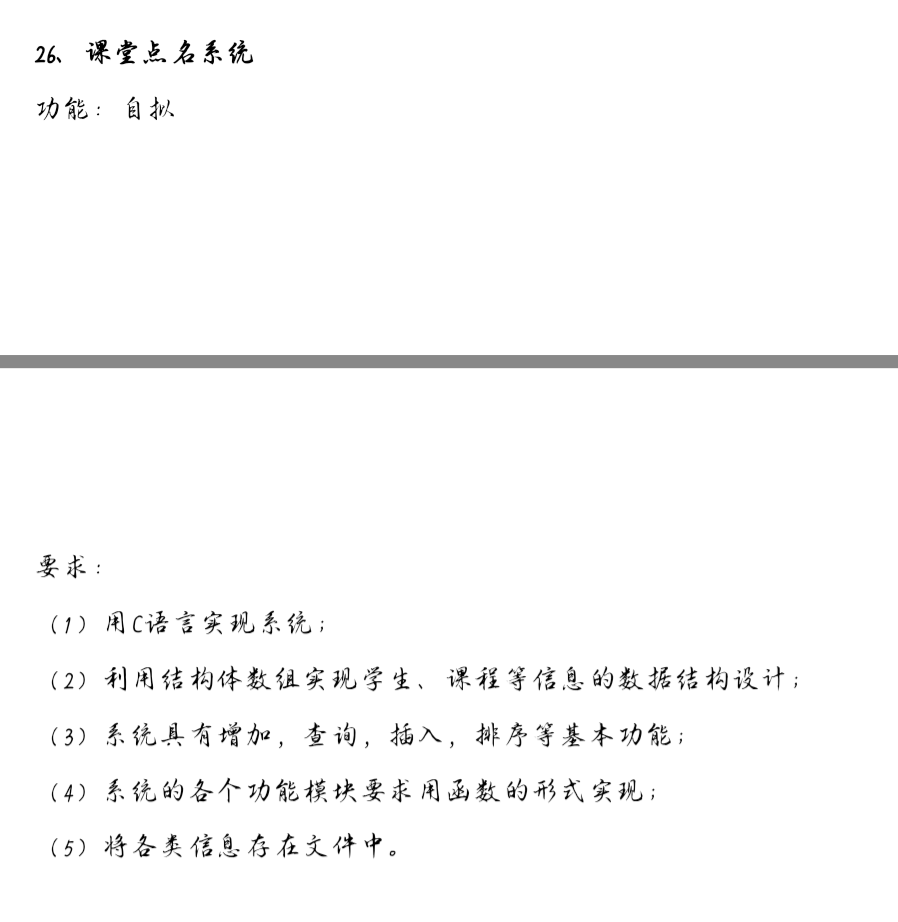2021-06-25 19:42

# 求一份C语言程序代码• 写回答
• 关注问题
• 收藏
• 邀请回答

#### 1条回答默认 最新

•无奈ieq 2021-06-25 20:10
已采纳
``````#include<stdio.h>
#include<malloc.h>
#include<stdlib.h>
#include<string.h>
typedef struct book{
int id;
char name;
int price;
char writer;
void show()    //展示
{
printf("名字：%s 编号：%d 价格：%d 作者：%s\n",name,id,price,writer);
}
struct book *next;
}book;

typedef struct library{
void find(char *NAME);   ///查找
void sdelete(char *NAME);   //删除
void show();  // 输出
void clear();  //内存回收
void Save();   //保存
void change(char *NAME);   //修改指定书本信息

}library;
//删除
void library::sdelete(char *NAME)
{
book *p1;
while(p)
{
if(strcmp((p->next)->name,NAME)==0)
{
p1=p->next;
p->next=p1->next;
free(p1);
printf("删除成功！") ;
break;
}
p=p->next;
if(p->next==NULL)
{
printf("查无此书！！请核对后重新尝试\n");
break;
}
}

}
//添加
{
book *p;
p=(book *)malloc(sizeof(book));
int ID;
char NAME;
int Price;
char Writer;
printf("请输入编号：");
scanf("%d",&ID);
p->id=ID;
printf("请输入书名：");
scanf("%s",NAME) ;
strcpy(p->name,NAME);
printf("请输入价格：");
scanf("%d",&Price) ;
p->price=Price;
printf("请输入作者：");
scanf("%s",&Writer);
strcpy(p->writer,Writer);
p->next=NULL;
return p;

}
//查找
void library::find(char* NAME)
{

while(p)
{
if(strcmp(p->name,NAME)==0)
{
printf("当前书本信息为：\n");
p->show();
break;
}
p=p->next;
}

if(p==NULL)
printf("查无此书！！请核对后重新尝试\n");

}
//显示所有书本信息
void library::show()
{
if(p==NULL)
printf("当前无信息！\n");
else
{
while(p)
{
p->show();
p=p->next;
}
}
}
//修改
void library::change(char *NAME)
{
p=p->next;
while(p)
{
if(strcmp(p->name,NAME)==0)
{
printf("请输入需要修改的信息：（1：价格 2：作者 ）");
int chose;
scanf("%d",&chose);
switch(chose)
{
case 1:
printf("请输入新价格：");
int a;
scanf("%d",&a);
p->price=a;
break;
case 2:
printf("请输入新作者：");
char w;
getchar();
gets(w);
strcpy(p->writer,w);
break;
default:
printf("输入错误！！");
}
}
break;
p=p->next;
}
if(p==NULL)
printf("未找到需要修改的书本！！！\n");
}

//内存回收
void library::clear()
{
while(p)
{
book *e=p;
p=e->next;
free(e);
}
}
//保存到文件
void library::Save()
{

FILE *fp;
fp=fopen("book.txt","w");
while(p0)
{
fprintf(fp,"%d %s %d %s",p0->id,p0->name,p0->price,p0->writer);
if(p0->next!=NULL)   //最后一组数据不换行避免出错
fprintf(fp,"\n",NULL);
p0=p0->next;
}
fclose(fp);
}
//从文件读到内存
{
FILE *fp;
fp=fopen("book.txt","a+");
fscanf(fp,"%d %s %d %s",&p->id,p->name,&p->price,p->writer);  //将第一组无用数据放到头结点
while(!feof(fp))
{
e=(book *)malloc(sizeof(book));
fscanf(fp,"%d %s %d %s",&e->id,e->name,&e->price,e->writer);
p->next=e;
p=p->next;
}
p->next=NULL;
fclose(fp);

}
int main()
{
library c;
printf("*                  图书信息管理系统                   *\n");
printf("*----------------------------------------------------------------*\n");
printf("*                          1/增加书籍信息                               *\n");
printf("*                          2/查询书籍信息                   *\n");
printf("*                          3/删除书籍信息                  *\n");
printf("*                          4/输出所有书籍信息                 *\n");
printf("*                          5/修改书籍信息                         *\n");
printf("*                         -1/退出 (否则无法保存)                           *\n");
printf("---------------------------------------------------------------------*\n");
int chose;
while(scanf("%d",&chose)!=-1)
{
system("cls");        //这部分用于清屏 并且能够看到功能及其选项
printf("*                  图书信息管理系统                   *\n");
printf("*----------------------------------------------------------------*\n");
printf("*                          1/增加书籍信息                               *\n");
printf("*                          2/查询书籍信息                   *\n");
printf("*                          3/删除书籍信息                  *\n");
printf("*                          4/输出所有书籍信息                 *\n");
printf("*                          5/修改书籍信息                         *\n");
printf("*                         -1/退出 (否则无法保存)                           *\n");
printf("---------------------------------------------------------------------*\n");
if(chose==-1)
break;
switch(chose)
{
case 1:
{
while(p1->next)
p1=p1->next;
printf("请继续选择服务\n");
break;
}
case 2:
char name;
printf("请输入书名：\n");
getchar();                      //吞掉一个回车键
gets(name);
c.find(name);
printf("请继续选择服务\n");
break;
case 3:
char name2;
printf("请输入书名：\n");
getchar();                         //吞掉一个回车键
gets(name2);
c.sdelete(name2);
printf("请继续选择服务\n");
break;
case 4:
c.show();
printf("请继续选择服务\n");
break;
case 5:
printf("请输入需要修改的书名：");
char name3;
getchar();
gets(name3);
c.change(name3);
printf("请继续选择服务\n");
break;
default:
printf("输入错误！！！请重新输入！！！\n");
}
}
printf("谢谢使用！！！\n");
c.Save();
c.clear();
return 0;
}``````

自己做的，你把对应的名字换下就行，可以的话采纳下

已采纳该答案
打赏 评论